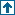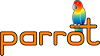parrotcode: Print the Mandelbrot set Contents | IMCC

# NAMEexamples/pir/mandel.pir - Print the Mandelbrot set

# SYNOPSIS`    % ./parrot examples/pir/mandel.pir`

# DESCRIPTIONThis prints an ASCII-art representation of the Mandelbrot set.

Translated from C code by Glenn Rhoads into Parrot assembler by Leon Brocard <acme@astray.com>. Translated from PASM to PIR by Bernhard Schmalhofer.

The C code is:

```    main() {
int x, y, k;
char *b = " .:,;!/>)|&IH%*#";
float r, i, z, Z, t, c, C;
for (y=30; puts(""), C = y*0.1 - 1.5, y--;) {
for (x=0; c = x*0.04 - 2, z=0, Z=0, x++ < 75;) {
for (r=c, i=C, k=0; t = z*z - Z*Z + r, Z = 2*z*Z + i, z=t, k<112; k++)
if (z*z + Z*Z > 10) break;
printf ("%c", b[k%16]);
}
}
}```